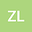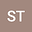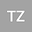Characteristics of the soliton solutions in a generalized variable-coefficient nonlinear Schr\”{o}dinger equation with single and double poles
•••• Zhi-Qiang Li,
• Shoufu Tian,
• TIan-Tian Zhang
Zhi-Qiang Li
China University of Mining and Technology
Author ProfileShoufu Tian
China University of Mining and Technology
Author ProfileTIan-Tian Zhang
China University of Mining and Technology
Author Profile## Abstract

A generalized variable-coefficient nonlinear Schr\”{o}dinger equation is investigated through the Riemann-Hilbert approach based on inverse scattering transformation with zero boundary conditions at infinity, and its various soliton solutions are successfully derived. To derive the eigenfunction and scattering matrix, and reveal their properties, the direct scattering problem is studied. Then based on inverse scattering transformation, a Riemann-Hilbert problem is constructed for the equation. For both cases of single and double poles, the Riemann-Hilbert problem is solved, and the formulae of soliton solutions are displayed. Finally, via evaluating the impact of each parameters, the soliton solutions are analyzed graphically involving $1$-, $2$- and $3$-soliton solutions.